Q

# Help me please, The three resistance of equal value are arranged in the different combinations shown below. Arrange them in increasing order of power dissipationI.II.III.IV.

The three resistance of equal value are arranged in the different combinations shown below. Arrange them in increasing order of power dissipation

I.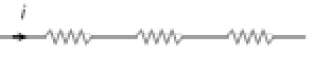II.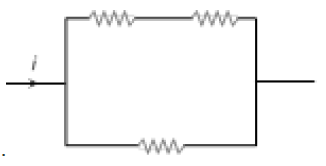III.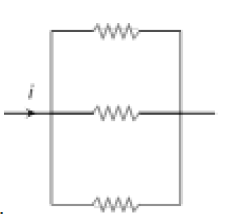IV.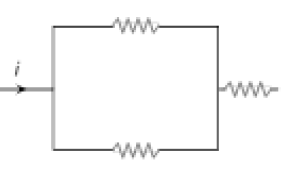• Option 1)

III < II < IV < I

• Option 2)

II < III < IV < I

• Option 3)

I < IV < III < II

• Option 4)

I < III < II < IV

239 Views

As we learnt in

Series Grouping -

Potential - Different

Current - Same

- wherein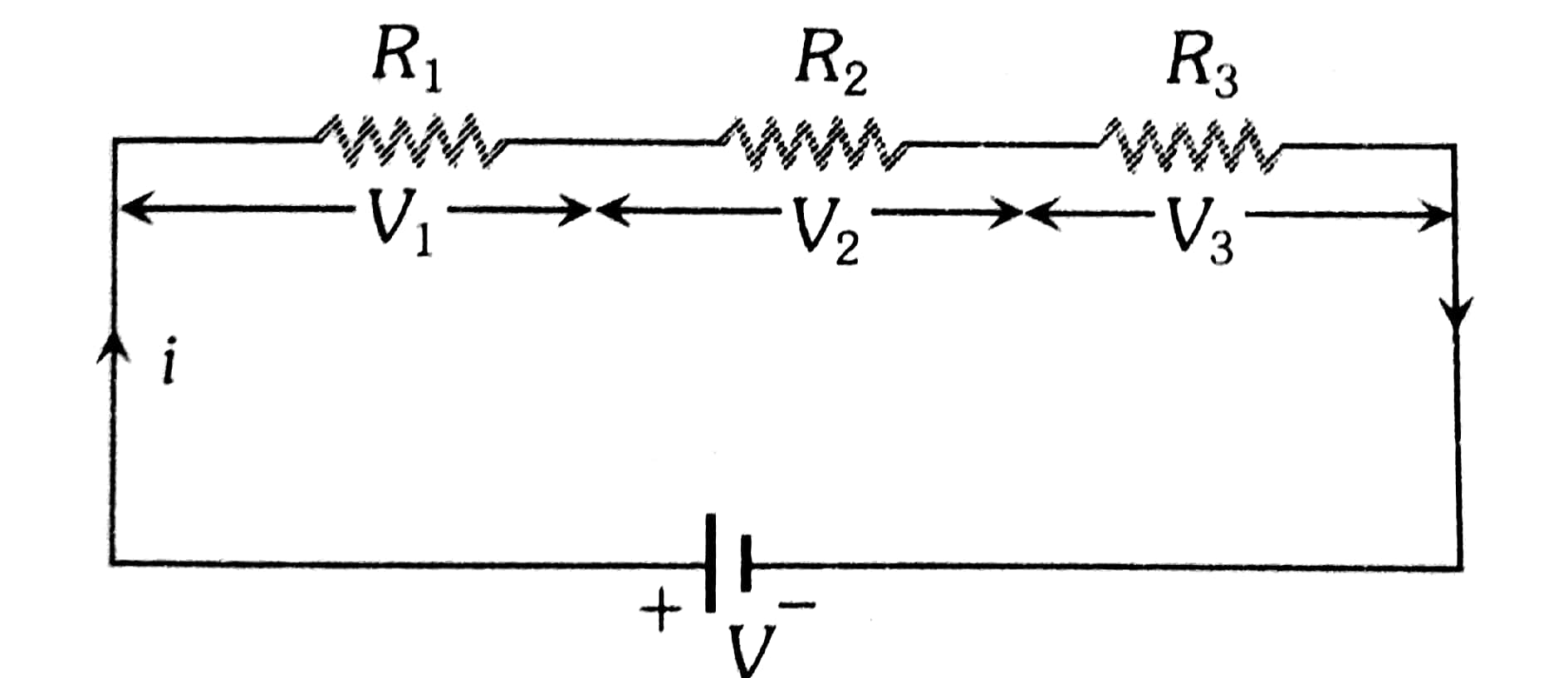&

Parallel Grouping -

Potential - Same

Current - Different

- wherein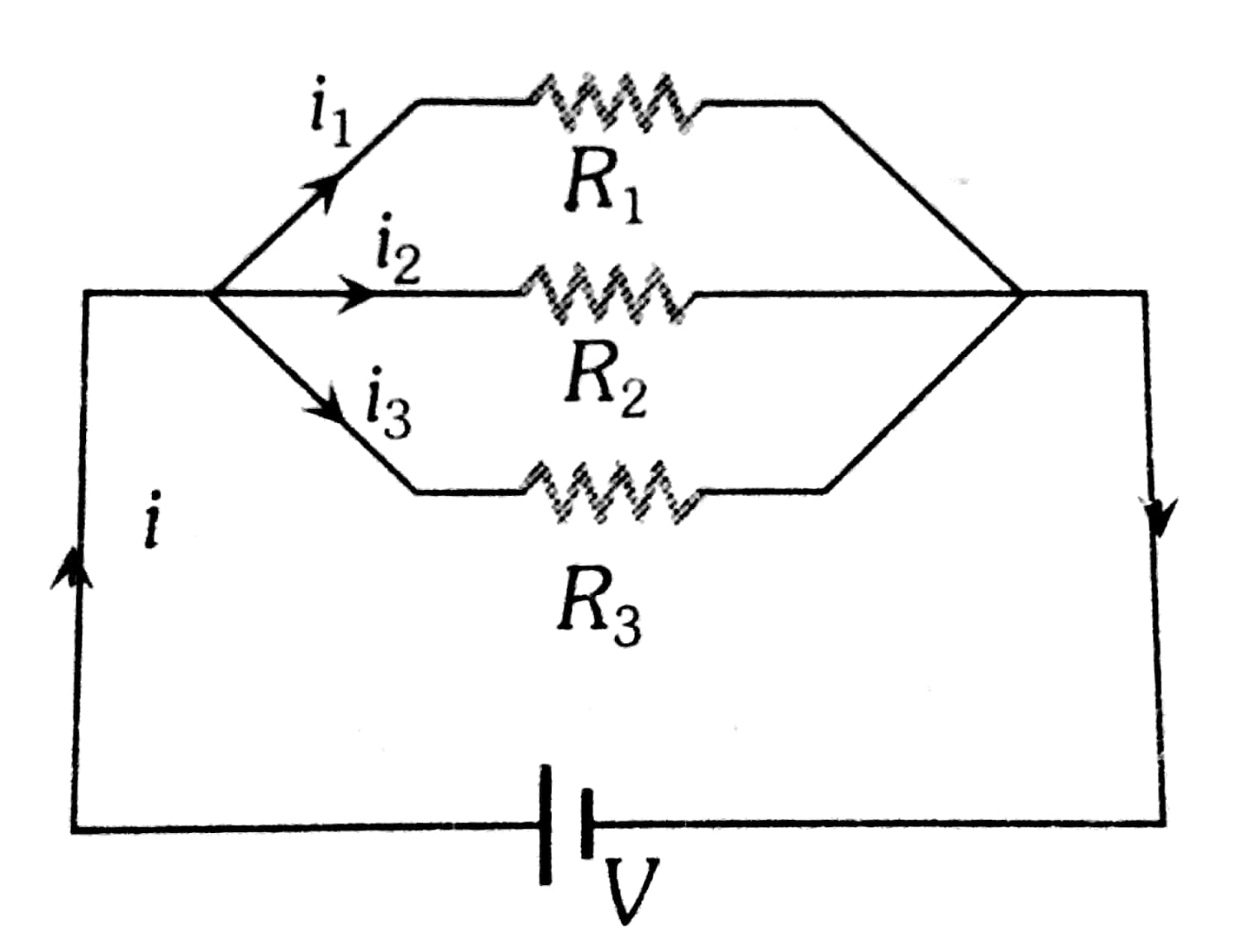P is maximum when R is

Order of power

iii <  ii < iv < i

Option 1)

III < II < IV < I

This solution is correct

Option 2)

II < III < IV < I

This solution is incorrect

Option 3)

I < IV < III < II

This solution is incorrect

Option 4)

I < III < II < IV

This solution is incorrect

Exams
Articles
Questions